Courses

# Organic Chemistry MCQ - 1 (Advanced)

## 28 Questions MCQ Test Chemistry for JEE Advanced | Organic Chemistry MCQ - 1 (Advanced)

Description
This mock test of Organic Chemistry MCQ - 1 (Advanced) for JEE helps you for every JEE entrance exam. This contains 28 Multiple Choice Questions for JEE Organic Chemistry MCQ - 1 (Advanced) (mcq) to study with solutions a complete question bank. The solved questions answers in this Organic Chemistry MCQ - 1 (Advanced) quiz give you a good mix of easy questions and tough questions. JEE students definitely take this Organic Chemistry MCQ - 1 (Advanced) exercise for a better result in the exam. You can find other Organic Chemistry MCQ - 1 (Advanced) extra questions, long questions & short questions for JEE on EduRev as well by searching above.
QUESTION: 1

### Which of (a)-(d) is the correct IUPAC name of the following compound?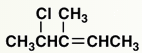Solution: When it loses proton/H+ nitrogen and oxygen gets negative charge and when u resonates the charges on any one of them u get same structures
QUESTION: 2

### Which of the following statements would be true about this compound: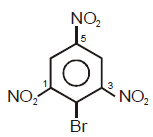Solution: There is an ortho effect on C1 and C3 N bonds. Due to ortho effect or SIR effect,there is no resonance between C1 NO2 and ring and also C3 NO2 and ring. So their bond character is single bond. In C5 N bond their is a double bond character due to its resonance with the ring. Since double bond length is smaller than single bond, So C is the answer.
QUESTION: 3

### Ease of ionization to produce carbocation and bromide ion under the treatment of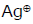will be maximum in which of the following compounds ?

Solution: OCH3 is ortho , para directing group. It increases electron density at para position , which stabilizes carbocation formed there. Hence C is the correct answer.
QUESTION: 4

Ease of ionization to produce carbocation and bromide ion under the treatment ofwill be maximum
in whichof the following compounds ?

Solution: Hybridization of the carbon is SP3 but the angle is 60 degree therefore the angle strain is very much hai so whenever this carbon gets a chance to reduce its angle strain try to reduce its hybridization so when the carbonation gets formed it's hybridization becomes SP2 so it will be the most fastest Processor it will remain in as ion as stable.
QUESTION: 5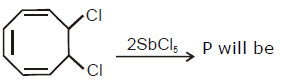Solution: The actual structure is not planar but if you consider it to make it aromatic to chlorine will be removed and two positive signs will occur it is called Quasi static compound in this compound 2 + ions will move inside the ring and form and aromatic system with 4 n+ 2 electrons that is six in this. Hence option B is correct answer.
QUESTION: 6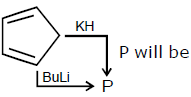Solution:
QUESTION: 7

Which one of the following statements is True:

(1)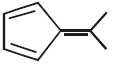(2 )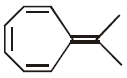Solution:
QUESTION: 8

Correct order of rate of hydrolysis or rate of reaction toward AgNO3 for following compounds is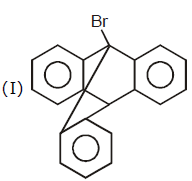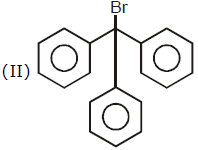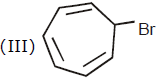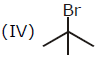Solution:
QUESTION: 9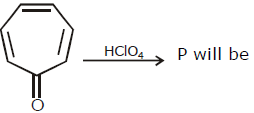Solution:
QUESTION: 10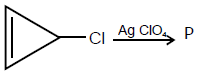Solution:
QUESTION: 11

Arrange the given phenols in their decreasing order of acidity: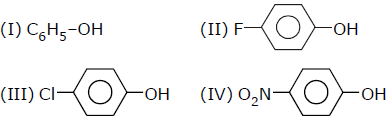Select the correct answer from the given code:

Solution:

IV will be most acidic owing to -M effect of -NO2. For comparison b/w halides, we use their +M effect(Just to check). F’s +M effect will be stronger than Cl’s as the F-C bond is shorter than the Cl-C bond.Also, electron density at F is greater than Cl. This will make electrons available to the acidic group thus decreasing its acidic strength. The last will be I. So the order is
IV>III>II>I.

QUESTION: 12

Which one of the following is the most acidic?

Solution:

Acidic nature can be calculated by the stability of conjugated base. That acid which makes more stable conjugated base is more stable. The leaving of H+ ion will form conjugate base having negative charge. By the concepts of aromaticity we come to know that option A and C will form anti aromatic compound. Option C will form compound having resonance but it will be anti-aromatic. But option B will form aromatic compound which is more stable than others. So B is most acidic having most stable conjugated base.

All finally comes down to the 4n + 2 rule.

Hence B

QUESTION: 13

Which of the following is most acidic

Solution:
m-chlorophenol is most acidic
alcohols are less acidic than phenol . Further Electron withdrawing group (Cl ) increases the acidity of phenol.
QUESTION: 14

Which of the following is weakest acid?

Solution:
QUESTION: 15

The correct pKa order of the follwoing acids is :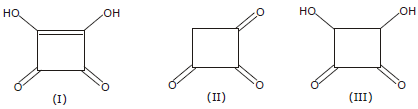Solution:

QUESTION: 16

Arrange pH of the given compounds in decreasing order:

(1) Phenol

(2) Ethyl alcohol
(3) Formic acid

(4) Benzoic acid

Solution:

pH of phenol, ethyl alcohol, formic acid and benzoic acid is 5-6, 7.33, 3.47 and 3.66 respectively

QUESTION: 17

Arrange acidity of given compounds in decreasing order: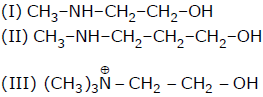Solution: As alkyl group increases +I effect increases more the -I effect more is acidity less the +I effect more is acidity.
*Multiple options can be correct
QUESTION: 18

Which of the above compounds reacts with NaHCO3 giving CO2

Solution:
*Multiple options can be correct
QUESTION: 19

Which of the following is a 2° Amine ?

Solution:
*Multiple options can be correct
QUESTION: 20

Identify electron - withdrawing groups in resonance among the following:

Solution:

Every carbonyl group are electron deficient and stablised by resonance. In cyanide nitrogen exhibiting -3 oxidation state and of its ionic character it also under goes resonance. except (e) all elements in given option undergoes resonance and are EWG.

*Multiple options can be correct
QUESTION: 21

Identify electron - donating groups in resonance among the following:

Solution:
*Multiple options can be correct
QUESTION: 22

In which of the following lone-pair indicated is involved in resonance :

Solution:

Resonance occurs in a conjugated system and in option b, d, e the lone pairs are joined in conjugation i.e. double bond then single bond and then lone pair. So, in these three cases the lone pair participates in resonance.

*Multiple options can be correct
QUESTION: 23

In which of the following lone-pair indicated is not involved in resonance :

Solution:
*Multiple options can be correct
QUESTION: 24

Aromatic compounds are:

Solution:

Aromatic compound formula is 4n + 2

where n = no. of π e's + lone pair e's whose orbital are parallel to adjacent C atom 's orbital

Non aromatic compound formula is 4n

In a,b ,d - n= 6 in each while in c option n= 4 as B has no lone pair of e's

QUESTION: 25

Identify the correct statement which is related to aromatic hydrocarbon?

Solution:

An aromatic hydrocarbon always has a sigma as well as a delocalized pi bond found between the carbon atoms.

*Multiple options can be correct
QUESTION: 26

Identify electron-donating groups in resonance among the following:

Solution:

Substituents with lone pairs (e.g. −OCH3, −NH2) on the atoms adjacent to the ππ-system are electron donating groups (EDG) in resonance systems. And, substituents with ππ-bonds to electronegative atoms (e.g. −C=O, -NO2) adjacent to the ππ-system are electron withdrawing groups (EWG) in a resonating structure

Therefore among the following groups electron-donating groups are:

(C) -OCOCH3, and (E) −NHCOCH3

*Multiple options can be correct
QUESTION: 27

Identify electron - withdrawing groups in resonance among the following:

Solution:

Substituents with lone pairs (e.g. −OCH3, −NH2) on the atoms adjacent to the π-system are electron donating groups (EDG) in resonance systems. And, substituents with ππ-bonds to electronegative atoms (e.g. −C=O, −NO2) adjacent to the π-system are electron withdrawing groups (EWG) in a resonating structure

Therefore among the following groups electron-donating groups are:

(C) −OCOCH3, and (E) −NHCOCH3

*Multiple options can be correct
QUESTION: 28

Which of the following reactions give aromatic compound ?

Solution: